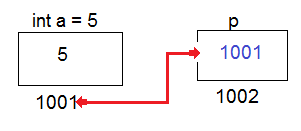Pointers In C

Usually variables stores values and values can be of any type (int,char,float etc).

Pointer is also a variable but it contains the address of any other variable.Representation Of Pointer In C. ByTechAchievers.com
here, "a" is the variable and it takes place in memory, assuming "a" stores in the 1001 address and "p" is the another variable holding a's location in memory called pointer.

Why We Use Pointers -
As we know that we can assign value to any variable in two ways,

1. provide constant value to the variable a = 5

2. ask value from user at run-time using scanf method.

but we are not able to change variable's value at run-time or dynamically, I have good news for you guys that we have pointer variables that can make changes in variable's value at run-time.

It is very much useful with data-structures like Linked List,Queue,Stack,Tree etc.

We can perform dynamic operations with variable's values, it reduce execution time and provides direct access to the variable.

How To Define Pointers -

Pointer variables are denoted by * (asterisk sign). Pointers will be declared as normal variable with proper data type.

As we learned that pointer can have direct access to another variable address and it can make any change to the variable's value, pointer datatype tells what kind of data type value will be effected by the pointer.

For example if pointer holds address of any int type variable then pointer datatype would be of int because pointer addressing to int variable.

It is practice to set pointer's default value as NULL.

Address of operator (&) tells the memory location of any variable, address is the hexadecimal value. To print hexadecimal we use %p format specifier.

In the example we have defined int pointer *A and assigning address of a variable by using & operator.

Memory address of a and value of pointer A are same.

When we write A then it shows value of pointer which is the memory address of variable but when we write *A that means we are pointing to int value of assigned address i.e 5

*A = 50, will change value at run-time, as you can see we didn't make change in a variable directly.

``````#include <stdio.h>

void  main() {

int a = 5;

int *A = NULL;

A = &a;

printf(" Value of a variable - %d  \n",a);

printf(" Memory address of a variable -  %p  \n",&a);

printf(" Pointer variable value - %p \n",A);

*A = 50;

printf(" Updated value of a variable - %d\n",a);

}

Output -

Value of a variable - 5

Memory address of a variable -  0x7fff8a89781c

Pointer variable value - 0x7fff8a89781c

Updated value of a variable - 50

``````

Different Ways To Use Pointers -

Pointers can be use anywhere in programs, it depends on program's requirement.
The key area of pointer is the direct access to any variable and it can access and change variable's value dynamically.

Let's check out all different scenarios in just one click given below
Different Ways To Use Pointers

1. Arithmetic operation with pointer

2. Pointer to pointer

3. Pointer with array

4. Pointer with functions

5. Pointer with strings

5. Pointer with structure